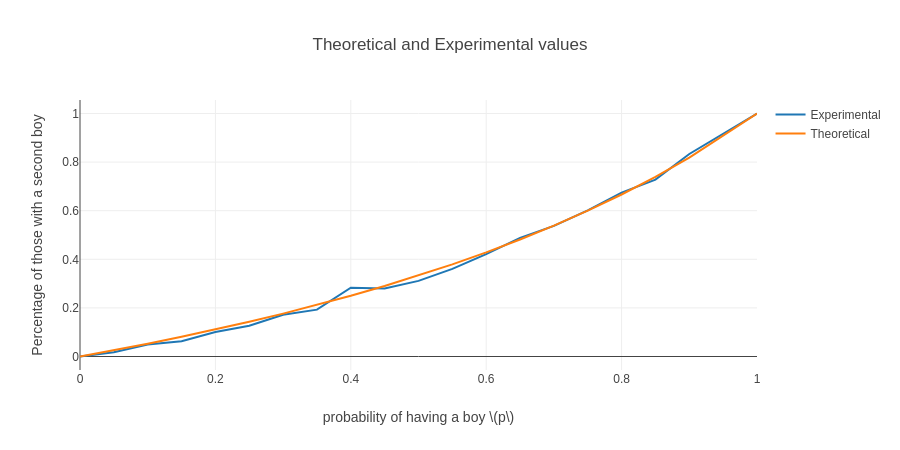# Boys in the hood

This post is really several riddles that get progressively more difficult. So don’t be dissuaded if you find the first few too easy (or if you don’t).

## Baby crazy

Let’s say we know a person named Pat who has two kids and at least one is a boy. Assuming that across the population the probability of having a boy is 50%. What is the probability that the other kid is a boy as well?

It’s 1/3 (or $33.\bar3$%). To see this, let’s just work out the possible states (the following states will be written as younger,older):

• B,B
• B,G
• G,B
• G,G

But since we know G,G is not a possibility (because Pat has at least one boy), and only one of the other three have the other child being a boy.

This might be easier to see and believe if you consider this using coin flips.

## To make things a little more difficult (as babies often do),

What’s the probability for an arbitrary probability $p$ of having a boy?

### Solution

We have the same states as before, but let’s first define some things to help us out:

\begin{align} BG=& \mathbb{P}(\text{boy})\cdot \mathbb{P}(\text{girl}) & GB=& \mathbb{P}(\text{girl})\cdot \mathbb{P}(\text{boy}) & BB=&\mathbb{P}(\text{boy})\cdot \mathbb{P}(\text{boy}) \\ =& p(1-p) & =& (1-p)p & =& p^2 \end{align}

now the probability is:

\begin{align} \frac{BB}{BG + GB + BB} =& \frac{p^2}{p(1-p) + (1-p)p + p^2} \\ =&\frac{p}{2(1-p)+p} = \frac{p}{2-p} \end{align}

In particular, if $p=0.5$, we get

\begin{align} \frac{p}{2(1-p)+p} = \frac{p}{2-p} =& \frac{0.5}{2-0.5} \\ \frac{0.5}{1.5} = \frac{1}{3} \end{align}

And just because I apparently like(?) making graphs…In case you’re eager to see the like 5 lines of code that went into making that graph, the notebook can be downloaded here.

## What if we know that the older child is a boy?

The whole paradoxical part of the problem goes away once we know the position of the known sex baby. By that I mean that the only possible states are:

• G,B
• B,B

So it’s 50/50.

## What if I told you that Pat has 2 kids, and one is a boy born in summer?

This is where it gets a little tricky. The states are now:

far too many to list. But, basically, it’s

$B\cdot{1 \text{ seasons}}, G\cdot{4 \text{ seasons}} \\ G\cdot{4 \text{ seasons}}, B\cdot{1 \text{ seasons}} \\ B\cdot{3 \text{ seasons}}, B\cdot{1 \text{ seasons}} \\ B\cdot{1 \text{ seasons}}, B\cdot{4 \text{ seasons}}$

Note that we’re not double counting the case where both are boys born in summer.

So there are a total of 15 possible outcomes, and 7 of them have a boy as the second child. So it’s $\frac{7}{15}$.

## What if we only know that one child is a boy born on December 23rd?

Or we could consider something a bit more general with probability $q$.

### Solution

We’re still assuming the probability of having a boy is $p$.

Then we can describe our exact table above like so:

\begin{align} \mathbb{P}(B\cdot{1 \text{ seasons}}, G\cdot{4 \text{ seasons}}) =& pq(1-p) & =& pq(1-p) \\ \mathbb{P}(G\cdot{4 \text{ seasons}}, B\cdot{1 \text{ seasons}}) =& (1-p)pq & =& pq(1-p) \\ \mathbb{P}(B\cdot{3 \text{ seasons}}, B\cdot{1 \text{ seasons}}) =& p(1-q)pq & =& p^2q(1-q) \\ \mathbb{P}(B\cdot{1 \text{ seasons}}, B\cdot{4 \text{ seasons}}) =& pqp & =& p^2q \end{align}

So we have:

\begin{align} \mathbb{P} =& \frac{p^2q(1-q) + p^2q}{p^2q+p^2q(1-q) + 2pq(1-p)} \\ =& \frac{pq\left(p(1-q) + p\right)}{pq\left(p+p(1-q)+2(1-p)\right)} \\ =& \frac{p(1-q) + p}{p+p(1-q)+2(1-p)} \\ =& \frac{p(2-q)}{2-pq} \end{align}

And we can see, as $q\to0$, $\mathbb{P}\to p$, and as $q\to1$, $\mathbb{P}\to \frac{p}{2-p}$

The closest thing I can give toward intuition here is this: let’s take the boy-in-summer example, and assume there’s a 25% chance of being born in summer. Then our universe of possibilities is restricting the known child to 1/4 of the possibilities otherwise, but there is no such restriction on the other child. So in that way, it’s in a sense down-weighting the effect of the known child (by scaling up all of the other possibilities).